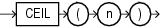## CEIL

SyntaxDescription of the illustration ceil.eps

Purpose

`CEIL` returns the smallest integer that is greater than or equal to `n`. The number `n` can always be written as the difference of an integer `k` and a positive fraction `f` such that 0 <= `f` < 1 and `n` = `k` - `f`. The value of `CEIL` is the integer `k`. Thus, the value of `CEIL` is `n` itself if and only if `n` is precisely an integer.

This function takes as an argument any numeric data type or any nonnumeric data type that can be implicitly converted to a numeric data type. The function returns the same data type as the numeric data type of the argument.

```SELECT order_total, CEIL(order_total)